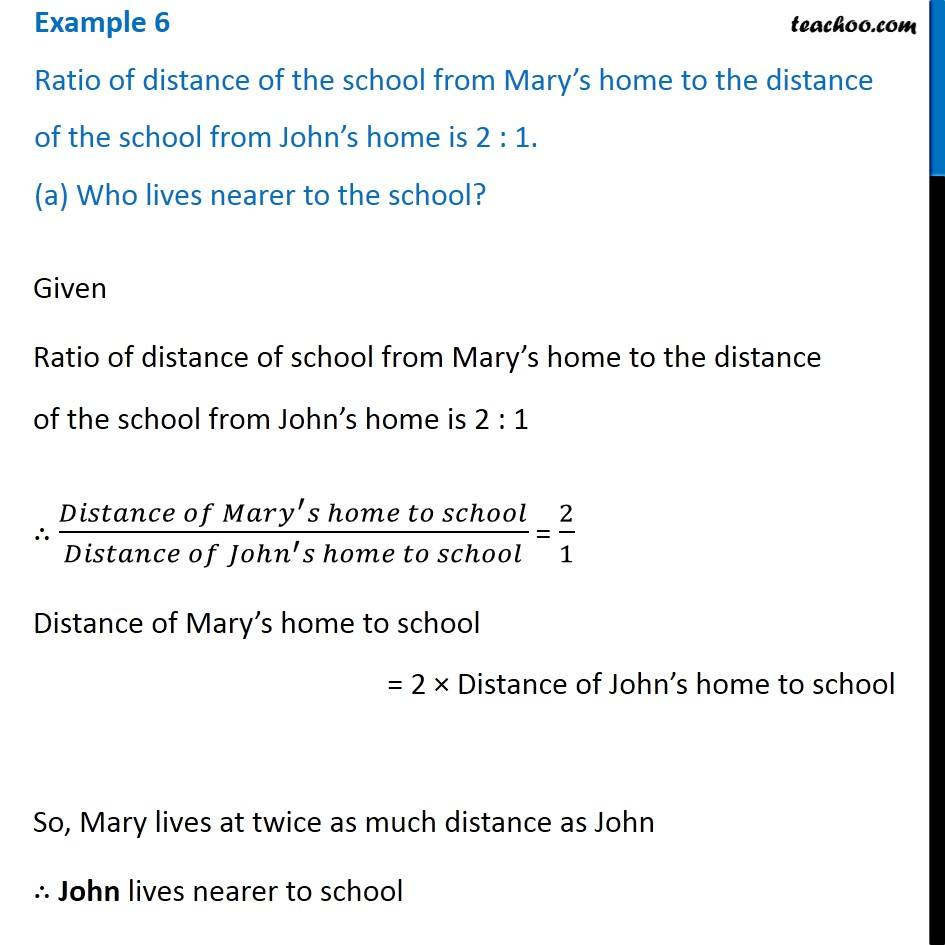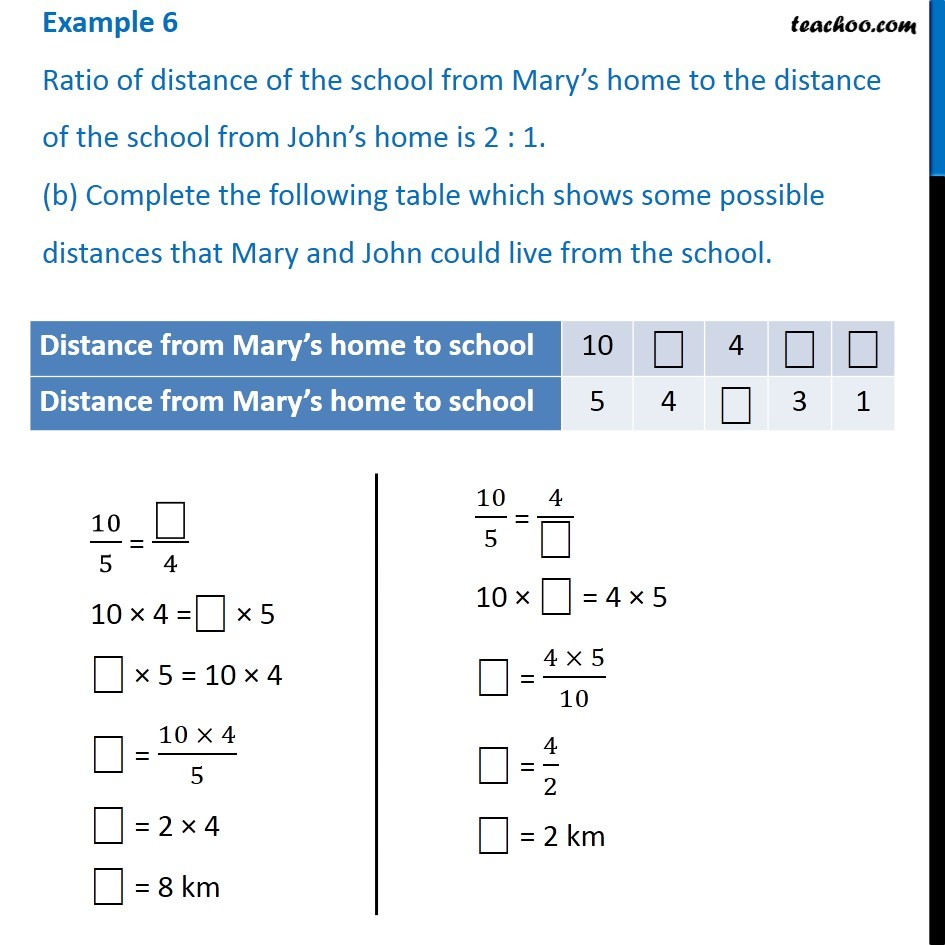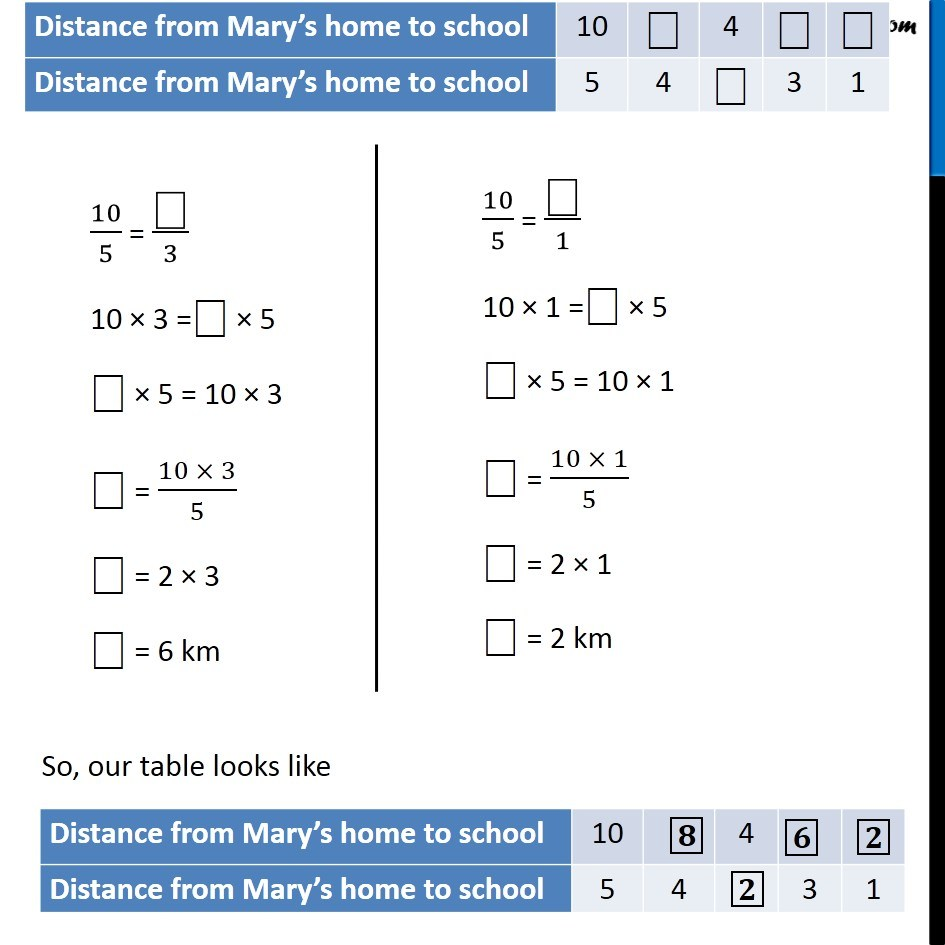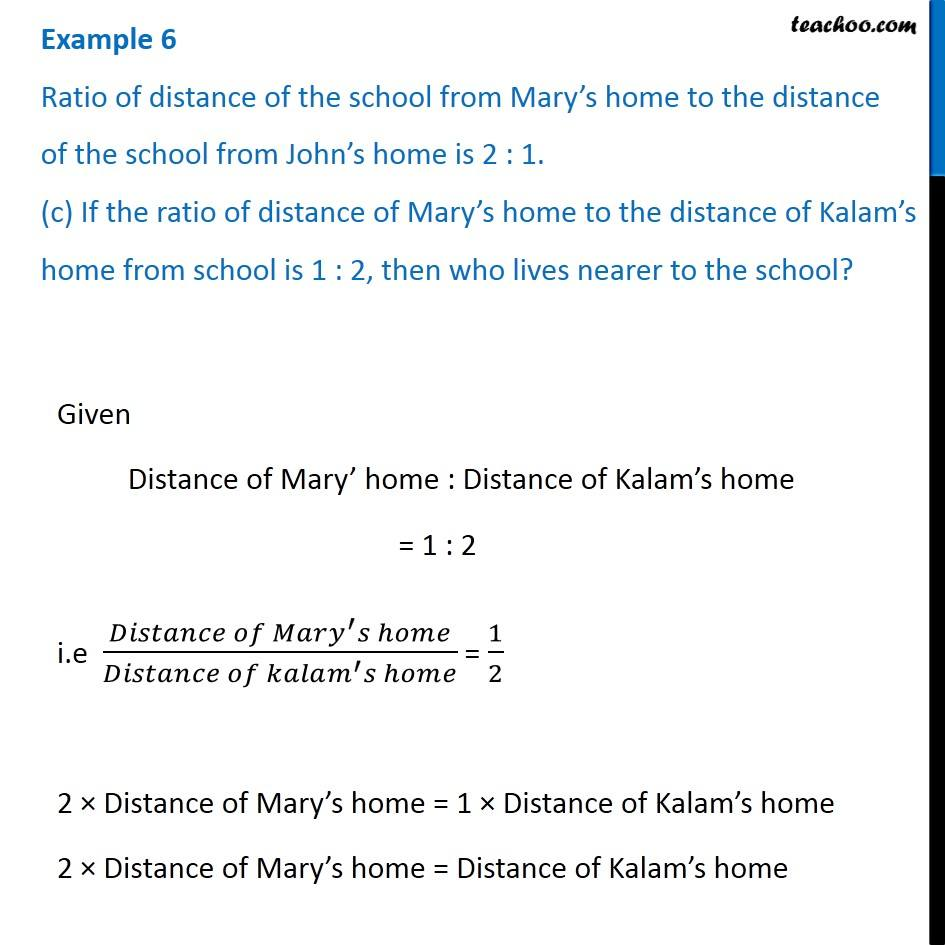Examples

Chapter 12 Class 6 Ratio And Proportion
Serial order wiseGet live Maths 1-on-1 Classs - Class 6 to 12

### Transcript

Example 6 - Chapter 12 Class 6 Ratio and Proportion - Class 6 Ratio of distance of the school from Mary’s home to the distance of the school from John’s home is 2 : 1. (a) Who lives nearer to the school? Given Ratio of distance of school from Mary’s home to the distance of the school from John’s home is 2 : 1 ∴ (𝐷𝑖𝑠𝑡𝑎𝑛𝑐𝑒 𝑜𝑓 𝑀𝑎𝑟𝑦^′ 𝑠 ℎ𝑜𝑚𝑒 𝑡𝑜 𝑠𝑐ℎ𝑜𝑜𝑙)/(𝐷𝑖𝑠𝑡𝑎𝑛𝑐𝑒 𝑜𝑓 𝐽𝑜ℎ𝑛^′ 𝑠 ℎ𝑜𝑚𝑒 𝑡𝑜 𝑠𝑐ℎ𝑜𝑜𝑙) = 2/1 Distance of Mary’s home to school = 2 × Distance of John’s home to school So, Mary lives at twice as much distance as John ∴ John lives nearer to school Example 6 Ratio of distance of the school from Mary’s home to the distance of the school from John’s home is 2 : 1. (b) Complete the following table which shows some possible distances that Mary and John could live from the school. 10/5 = "⎕" /4 10 × 4 ="⎕" × 5 "⎕" × 5 = 10 × 4 "⎕" = (10 × 4)/5 "⎕" = 2 × 4 "⎕" = 8 km 10/5 = 4/"⎕" 10 × "⎕" = 4 × 5 "⎕" = (4 × 5)/10 "⎕" = 4/2 "⎕" = 2 km 10/5 = "⎕" /3 10 × 3 ="⎕" × 5 "⎕" × 5 = 10 × 3 "⎕" = (10 × 3)/5 "⎕" = 2 × 3 "⎕" = 6 km 10/5 = "⎕" /1 10 × 1 ="⎕" × 5 "⎕" × 5 = 10 × 1 "⎕" = (10 × 1)/5 "⎕" = 2 × 1 "⎕" = 2 km Example 6 Ratio of distance of the school from Mary’s home to the distance of the school from John’s home is 2 : 1. (c) If the ratio of distance of Mary’s home to the distance of Kalam’s home from school is 1 : 2, then who lives nearer to the school? Given Distance of Mary’ home : Distance of Kalam’s home = 1 : 2 i.e (𝐷𝑖𝑠𝑡𝑎𝑛𝑐𝑒 𝑜𝑓 𝑀𝑎𝑟𝑦^′ 𝑠 ℎ𝑜𝑚𝑒)/(𝐷𝑖𝑠𝑡𝑎𝑛𝑐𝑒 𝑜𝑓 𝑘𝑎𝑙𝑎𝑚^′ 𝑠 ℎ𝑜𝑚𝑒) = 1/2 2 × Distance of Mary’s home = 1 × Distance of Kalam’s home 2 × Distance of Mary’s home = Distance of Kalam’s home ∴ Kalam’s home is twice as far as Mary’s home from school So, Mary lives nearer to school